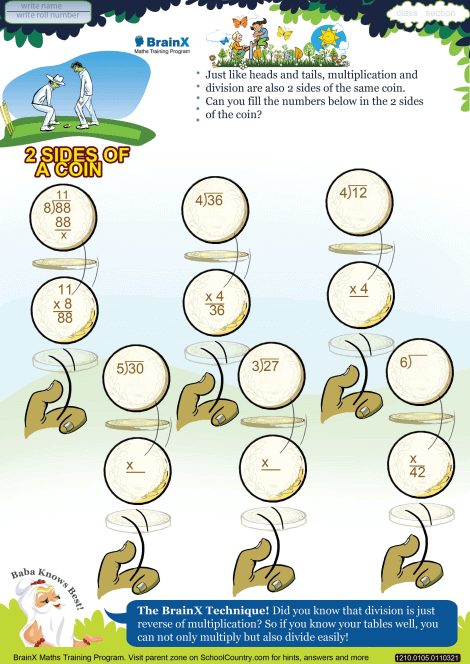# 2 sides of coin Worksheet Mark to Download Later

1 RatingsSelect Worksheet Theme to Download:

## About 2 sides of coin Worksheet

Multiplication is reverse of division. If we multiply the quotient and divisor, provide remainder is zero, we get the dividend. ->So by remembering the multiplication tables one can easily do division and multiplication

### Math Skills

• Division Facts when Dividend is of 2 Digit and Divisor of 1 Digit without remainder-Dividend upto 99

### Life Skills

• Memory - Recall, Speed
• Fluid intelligence - observation